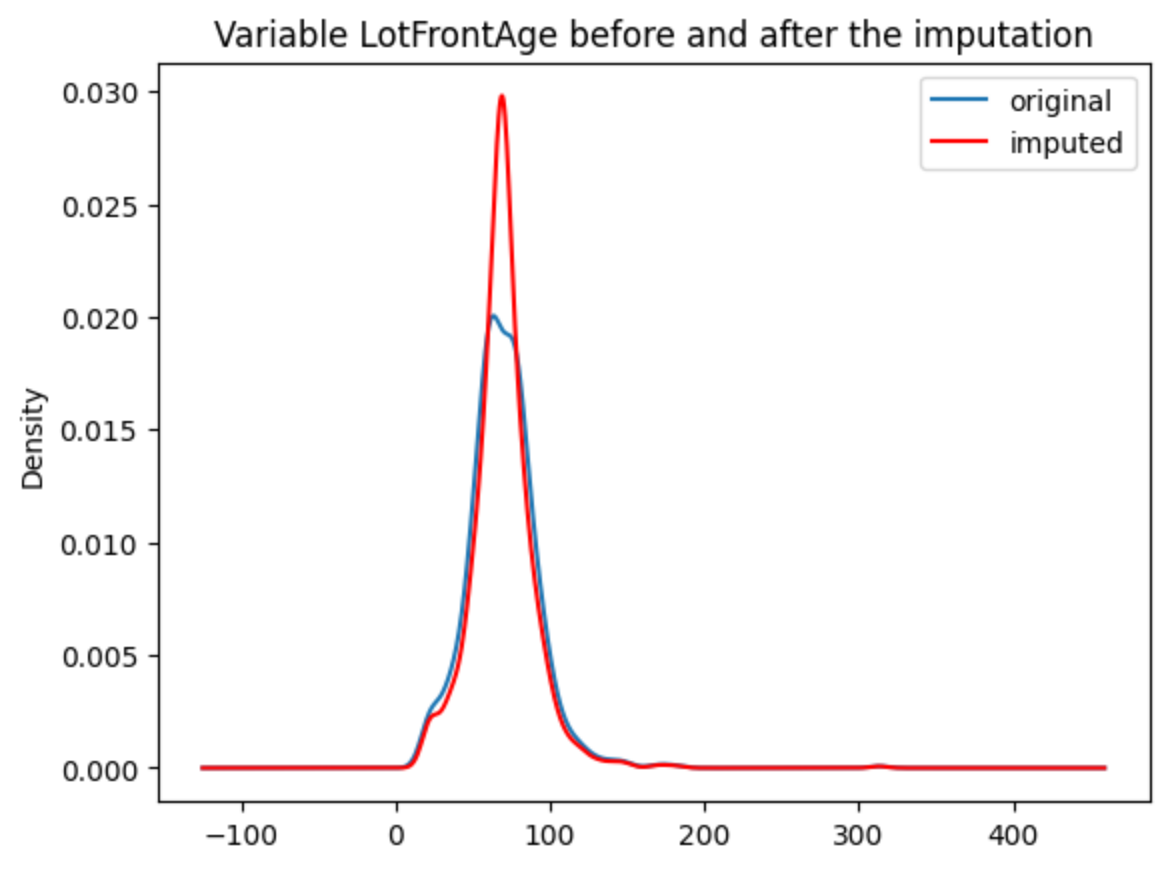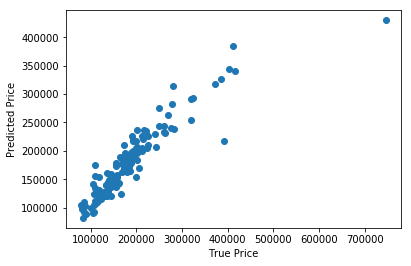# Quick Start#

If you’re new to Feature-engine this guide will get you started. Feature-engine transformers have the methods `fit()` and `transform()` to learn parameters from the data and then modify the data. They work just like any Scikit-learn transformer.

## Installation#

Feature-engine is a Python 3 package and works well with 3.7 or later. Earlier versions are not compatible with the latest versions of Python numerical computing libraries.

```\$ pip install feature-engine
```

Note, you can also install it with a _ as follows:

```\$ pip install feature_engine
```

Note that Feature-engine is an active project and routinely publishes new releases. In order to upgrade Feature-engine to the latest version, use `pip` as follows.

```\$ pip install -U feature-engine
```

If you’re using Anaconda, you can install the Anaconda Feature-engine package:

```\$ conda install -c conda-forge feature_engine
```

Once installed, you should be able to import Feature-engine without an error, both in Python and in Jupyter notebooks.

## Example Use#

This is an example of how to use Feature-engine’s transformers to perform missing data imputation.

```import numpy as np
import pandas as pd
import matplotlib.pyplot as plt
from sklearn.model_selection import train_test_split

from feature_engine.imputation import MeanMedianImputer

# Separate into train and test sets
X_train, X_test, y_train, y_test = train_test_split(
data.drop(['Id', 'SalePrice'], axis=1),
data['SalePrice'],
test_size=0.3,
random_state=0
)

# set up the imputer
median_imputer = MeanMedianImputer(
imputation_method='median', variables=['LotFrontage', 'MasVnrArea']
)

# fit the imputer
median_imputer.fit(X_train)

# transform the data
train_t = median_imputer.transform(X_train)
test_t = median_imputer.transform(X_test)

fig = plt.figure()
X_train['LotFrontage'].plot(kind='kde', ax=ax)
train_t['LotFrontage'].plot(kind='kde', ax=ax, color='red')
lines, labels = ax.get_legend_handles_labels()
ax.legend(lines, labels, loc='best')
```## Feature-engine with the Scikit-learn’s pipeline#

Feature-engine’s transformers can be assembled within a Scikit-learn pipeline. This way, we can store our entire feature engineering pipeline in one single object or pickle (.pkl). Here is an example of how to do it:

```from math import sqrt
import pandas as pd
import numpy as np
import matplotlib.pyplot as plt

from sklearn.linear_model import Lasso
from sklearn.metrics import mean_squared_error
from sklearn.model_selection import train_test_split
from sklearn.pipeline import Pipeline as pipe
from sklearn.preprocessing import MinMaxScaler

from feature_engine.encoding import RareLabelEncoder, MeanEncoder
from feature_engine.discretisation import DecisionTreeDiscretiser
from feature_engine.imputation import (
MeanMedianImputer,
CategoricalImputer,
)

# drop some variables
data.drop(
axis=1,
inplace=True
)

# make a list of categorical variables
categorical = [var for var in data.columns if data[var].dtype == 'O']

# make a list of numerical variables
numerical = [var for var in data.columns if data[var].dtype != 'O']

# make a list of discrete variables
discrete = [ var for var in numerical if len(data[var].unique()) < 20]

# categorical encoders work only with object type variables
# to treat numerical variables as categorical, we need to re-cast them
data[discrete]= data[discrete].astype('O')

# continuous variables
numerical = [
var for var in numerical if var not in discrete
and var not in ['Id', 'SalePrice']
]

# separate into train and test sets
X_train, X_test, y_train, y_test = train_test_split(
data.drop(labels=['SalePrice'], axis=1),
data.SalePrice,
test_size=0.1,
random_state=0
)

# set up the pipeline
price_pipe = pipe([
# add a binary variable to indicate missing information for the 2 variables below

# replace NA by the median in the 2 variables below, they are numerical
('continuous_var_median_imputer', MeanMedianImputer(
imputation_method='median', variables=['LotFrontage', 'MasVnrArea']
)),

# replace NA by adding the label "Missing" in categorical variables
('categorical_imputer', CategoricalImputer(variables=categorical)),

# disretise continuous variables using trees
('numerical_tree_discretiser', DecisionTreeDiscretiser(
cv=3,
scoring='neg_mean_squared_error',
variables=numerical,
regression=True)),

# remove rare labels in categorical and discrete variables
('rare_label_encoder', RareLabelEncoder(
tol=0.03, n_categories=1, variables=categorical+discrete
)),

# encode categorical and discrete variables using the target mean
('categorical_encoder', MeanEncoder(variables=categorical+discrete)),

# scale features
('scaler', MinMaxScaler()),

# Lasso
('lasso', Lasso(random_state=2909, alpha=0.005))

])

# train feature engineering transformers and Lasso
price_pipe.fit(X_train, np.log(y_train))

# predict
pred_train = price_pipe.predict(X_train)
pred_test = price_pipe.predict(X_test)

# Evaluate
print('Lasso Linear Model train mse: {}'.format(
mean_squared_error(y_train, np.exp(pred_train))))
print('Lasso Linear Model train rmse: {}'.format(
sqrt(mean_squared_error(y_train, np.exp(pred_train)))))
print()
print('Lasso Linear Model test mse: {}'.format(
mean_squared_error(y_test, np.exp(pred_test))))
print('Lasso Linear Model test rmse: {}'.format(
sqrt(mean_squared_error(y_test, np.exp(pred_test)))))
```
```Lasso Linear Model train mse: 949189263.8948538
Lasso Linear Model train rmse: 30808.9153313591

Lasso Linear Model test mse: 1344649485.0641894
Lasso Linear Model train rmse: 36669.46256852136
```
```plt.scatter(y_test, np.exp(pred_test))
plt.xlabel('True Price')
plt.ylabel('Predicted Price')
plt.show()
```### More examples#

More examples can be found in: